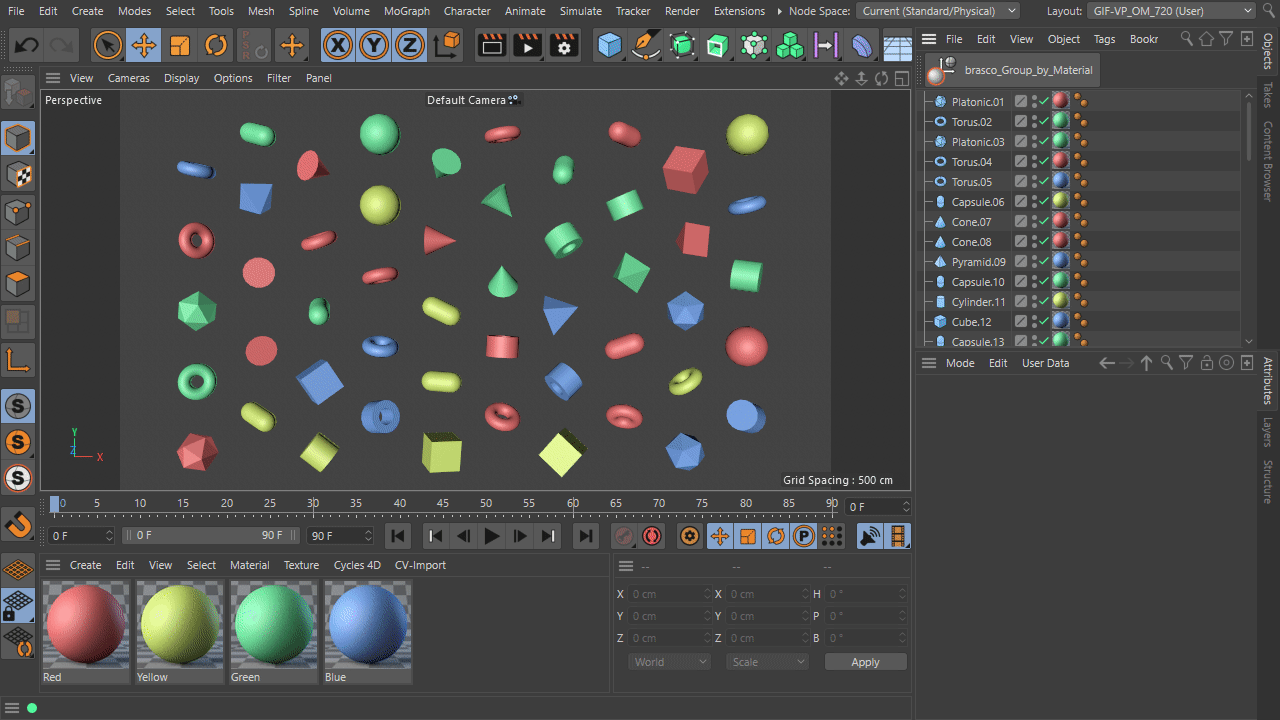# Python script: about select material then group objects

#1

Hi~
i want to write a script ,like this
select a material which name is “red” , then use command “Select Material Tags/Objects” to select objects, then group this objects,and rename the group “red”
i find a lot tutorial but still don’t understand
hope someone can help me, thanks a lot!

#2

Hi,

Yes, you can do this by getting the Active Material’s MatAssignData and building a list of the objects that it is assigned to.

Beyond the basics of Getting the active material (we’ll use “mat” in the examples), you want to then get the MatAssignData of said material using:

``````matAssignData = mat[c4d.ID_MATERIALASSIGNMENTS]
``````

From that you can get the count of objects in that assignment list:

``````count = matAssignData.GetCount()
``````

You can then use this to iterate over the items in the material’s assignement data and get the tags and from the tag, the object:

``````for i in range(count):

tag = matAssignData.ObjectFromIndex(doc,i)
obj = tag.GetObject()
``````

That is the basics for getting the objects in the selected material’s assignment list.

Here is that implemented in a script I wrote (modified from one that does something a bit similar) for you to reverse engineer:wow! thank you very much!